# Joel Lewis - MIT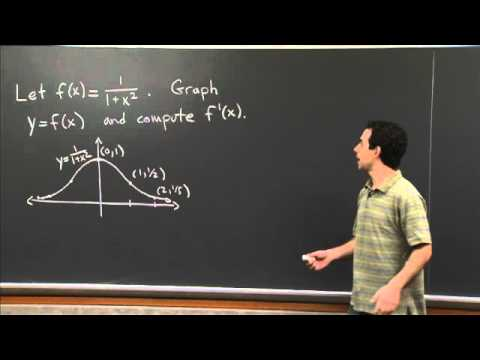#### Definition of the Derivative | MIT 18.01SC Single Variable Calculus, Fall 2010

Topic: Calculus
Teacher: Joel Lewis
Concepts In This Lecture: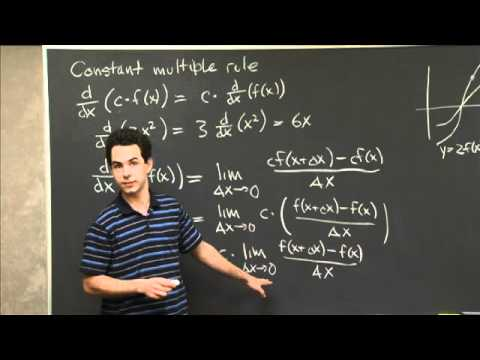#### Constant Multiple Rule | MIT 18.01SC Single Variable Calculus, Fall 2010

Topic: Calculus
Teacher: Joel Lewis
Concepts In This Lecture: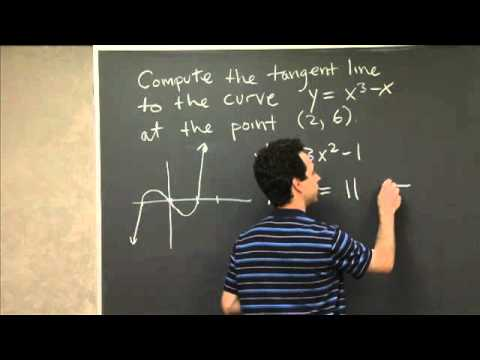#### Tangent Line to a Polynomial | MIT 18.01SC Single Variable Calculus, Fall 2010

Topic: Calculus
Teacher: Joel Lewis
Concepts In This Lecture: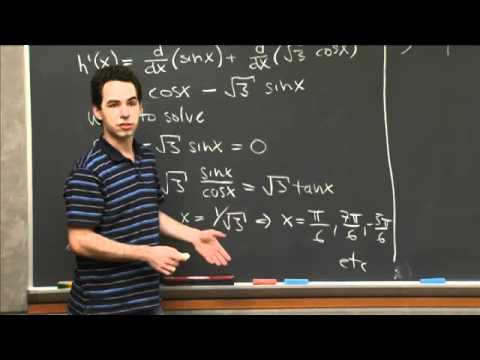#### Derivatives of Sine and Cosine | MIT 18.01SC Single Variable Calculus, Fall 2010

Topic: Calculus
Teacher: Joel Lewis
Concepts In This Lecture: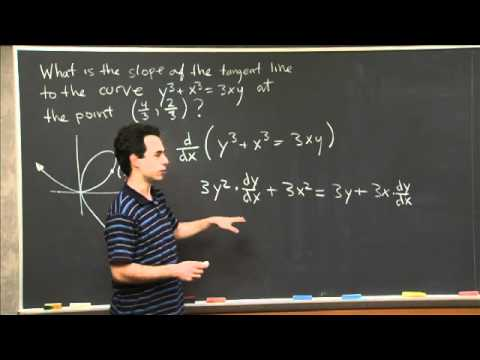#### Implicit Differentiation | MIT 18.01SC Single Variable Calculus, Fall 2010

Topic: Calculus
Teacher: Joel Lewis
Concepts In This Lecture: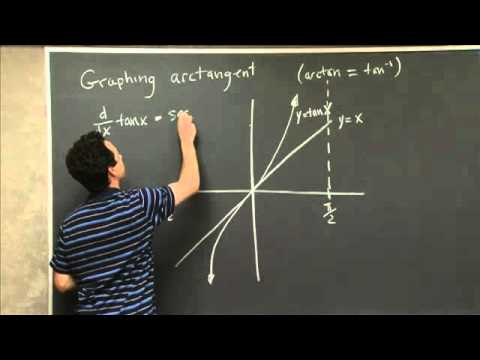#### Graphing the Arctan Function | MIT 18.01SC Single Variable Calculus, Fall 2010

Topic: Calculus
Teacher: Joel Lewis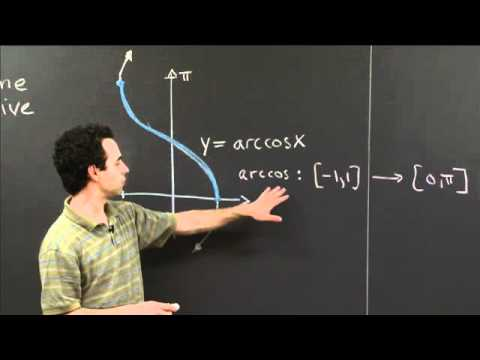#### Arccos | MIT 18.01SC Single Variable Calculus, Fall 2010

Topic: Calculus
Teacher: Joel Lewis
Concepts In This Lecture: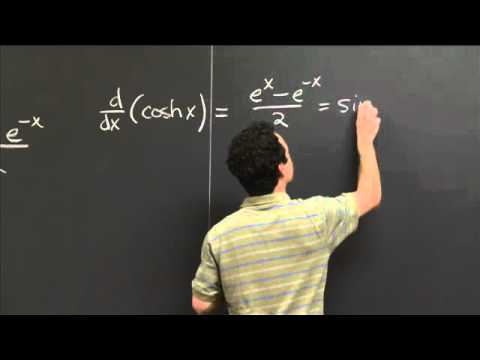#### Hyperbolic trig functions | MIT 18.01SC Single Variable Calculus, Fall 2010

Topic: Calculus
Teacher: Joel Lewis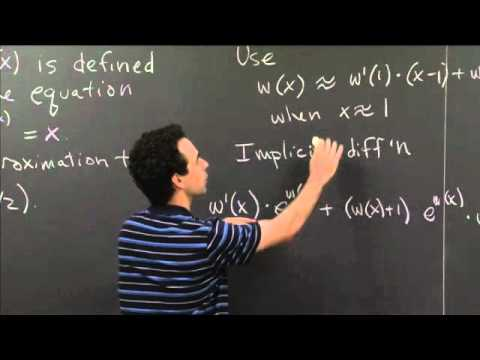#### Implicit Differentiation and Linear Approximation | MIT 18.01SC Single Variable Calculus, Fall 2010

Topic: Calculus
Teacher: Joel Lewis
Concepts In This Lecture: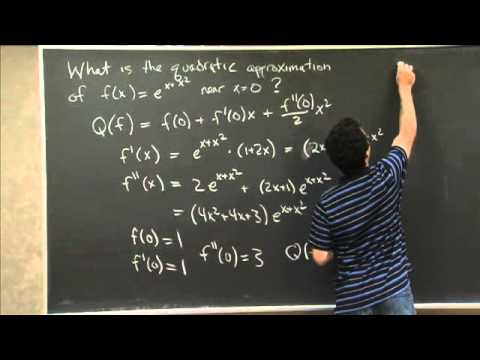#### Quadratic Approximation | MIT 18.01SC Single Variable Calculus, Fall 2010

Topic: Calculus
Teacher: Joel Lewis
Concepts In This Lecture: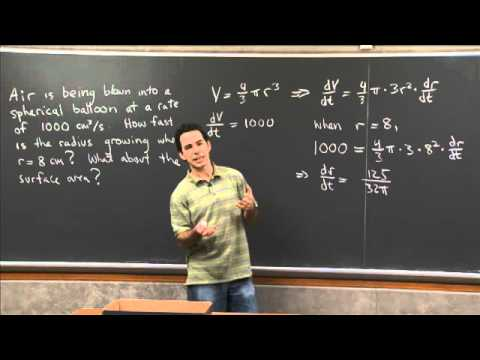#### Related rates 1 | MIT 18.01SC Single Variable Calculus, Fall 2010

Topic: Calculus
Teacher: Joel Lewis
Concepts In This Lecture: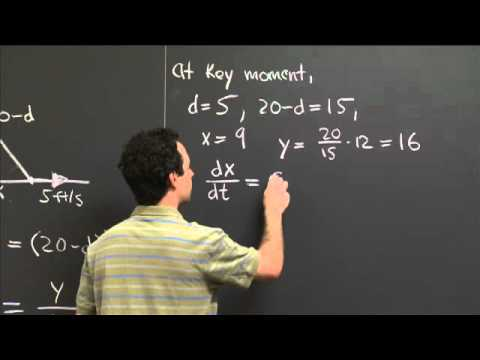#### Related rates 2 | MIT 18.01SC Single Variable Calculus, Fall 2010

Topic: Calculus
Teacher: Joel Lewis
Concepts In This Lecture: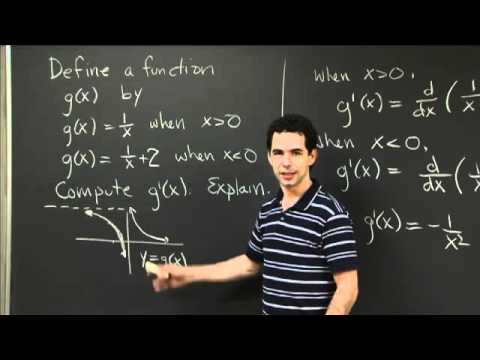#### Antidiff. With Discontinuity | MIT 18.01SC Single Variable Calculus, Fall 2010

Topic: Calculus
Teacher: Joel Lewis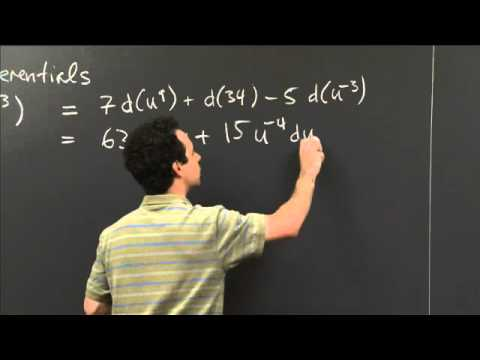#### Computing Differentials | MIT 18.01SC Single Variable Calculus, Fall 2010

Topic: Calculus
Teacher: Joel Lewis
Concepts In This Lecture: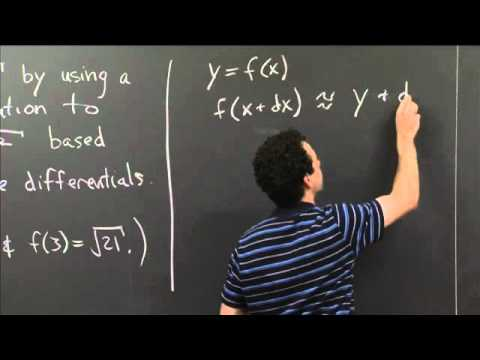#### Linear approx. with differentials | MIT 18.01SC Single Variable Calculus, Fall 2010

Topic: Calculus
Teacher: Joel Lewis
Concepts In This Lecture: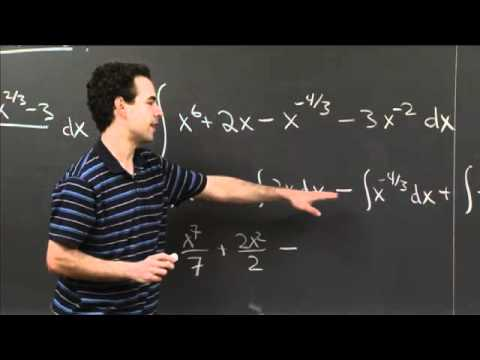#### Computing Antiderivatives | MIT 18.01SC Single Variable Calculus, Fall 2010

Topic: Calculus
Teacher: Joel Lewis
Concepts In This Lecture: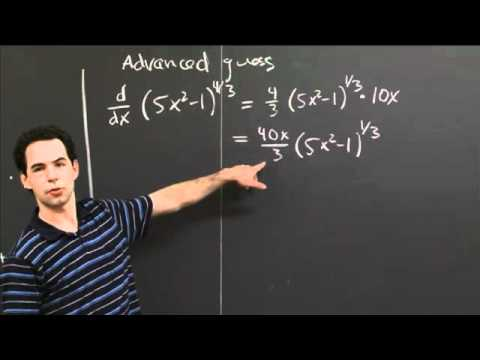#### Antidifferentiation by substitution | MIT 18.01SC Single Variable Calculus, Fall 2010

Topic: Calculus
Teacher: Joel Lewis
Concepts In This Lecture: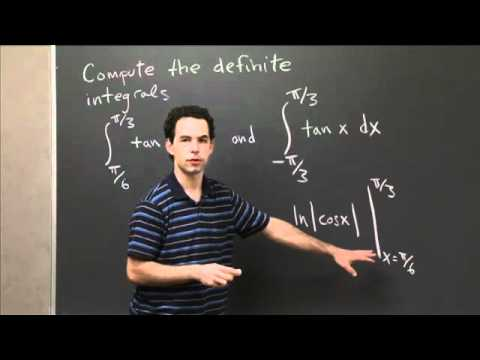#### Definite Integrals of tan(x) | MIT 18.01SC Single Variable Calculus, Fall 2010

Topic: Calculus
Teacher: Joel Lewis
Concepts In This Lecture: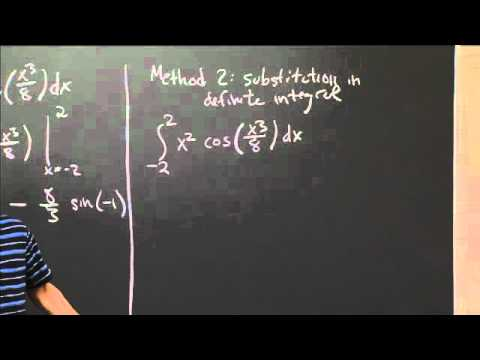#### Definite Integral by Substitution | MIT 18.01SC Single Variable Calculus, Fall 2010

Topic: Calculus
Teacher: Joel Lewis
Concepts In This Lecture: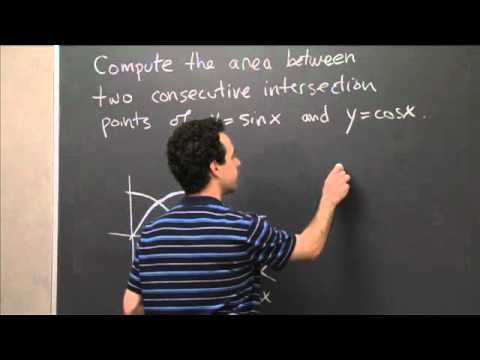#### Area Between the Graphs of Sine and Cosine | MIT 18.01SC Single Variable Calculus, Fall 2010

Topic: Calculus
Teacher: Joel Lewis
Concepts In This Lecture: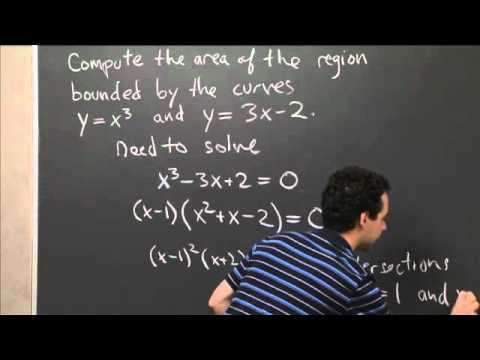#### Area Between y=x^3 and y=3x-2 | MIT 18.01SC Single Variable Calculus, Fall 2010

Topic: Calculus
Teacher: Joel Lewis
Concepts In This Lecture: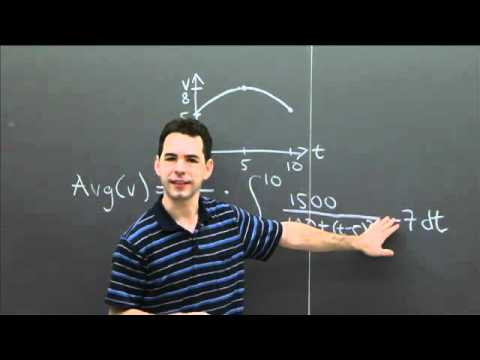#### Average Velocity | MIT 18.01SC Single Variable Calculus, Fall 2010

Topic: Calculus
Teacher: Joel Lewis
Concepts In This Lecture: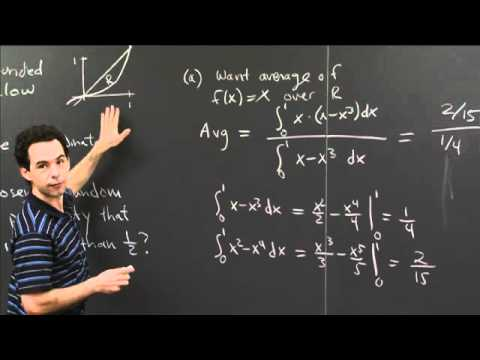#### Average x-Coordinate in a Region | MIT 18.01SC Single Variable Calculus, Fall 2010

Topic: Calculus
Teacher: Joel Lewis
Concepts In This Lecture: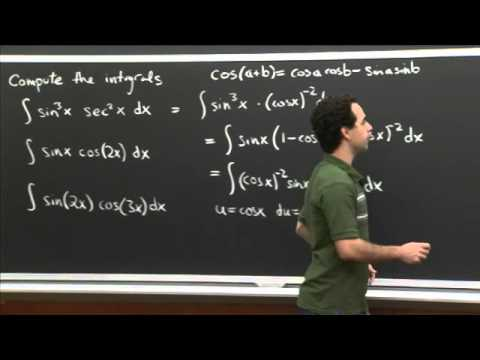#### Trig Integral Practice | MIT 18.01SC Single Variable Calculus, Fall 2010

Topic: Calculus
Teacher: Joel Lewis
Concepts In This Lecture: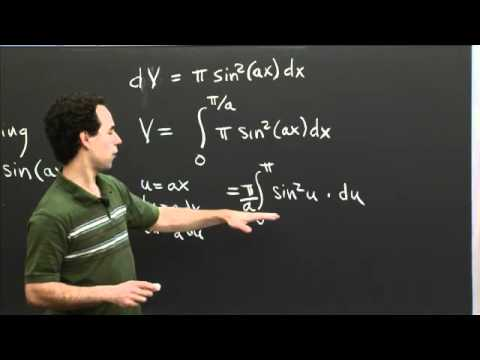#### Trig Integrals and a Volume of Revolution | MIT 18.01SC Single Variable Calculus, Fall 2010

Topic: Calculus
Teacher: Joel Lewis
Concepts In This Lecture: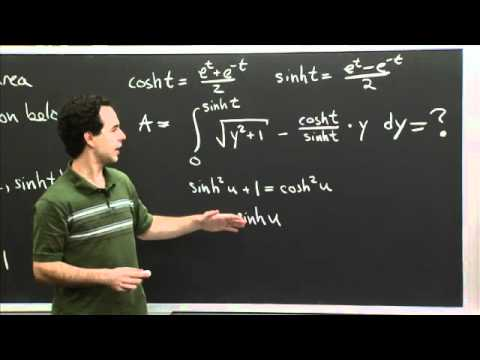#### Hyperbolic Trig Sub | MIT 18.01SC Single Variable Calculus, Fall 2010

Topic: Calculus
Teacher: Joel Lewis
Concepts In This Lecture: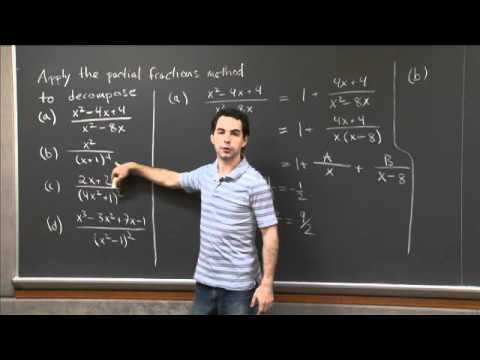#### Partial Fractions Decomposition | MIT 18.01SC Single Variable Calculus, Fall 2010

Topic: Calculus
Teacher: Joel Lewis
Concepts In This Lecture: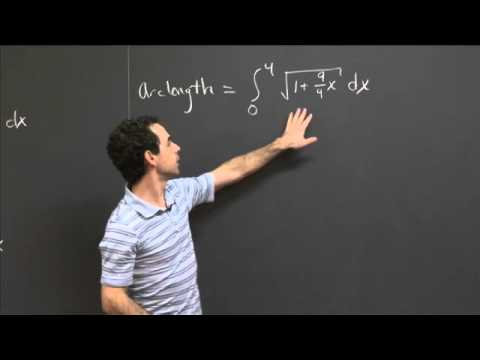#### Arc Length of y=x^(2/3) | MIT 18.01SC Single Variable Calculus, Fall 2010

Topic: Calculus
Teacher: Joel Lewis
Concepts In This Lecture: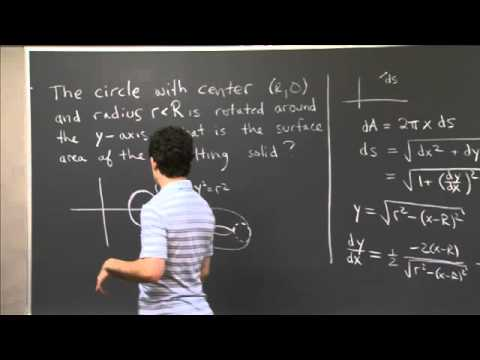#### Surface Area of a Torus | MIT 18.01SC Single Variable Calculus, Fall 2010

Topic: Calculus
Teacher: Joel Lewis
Concepts In This Lecture: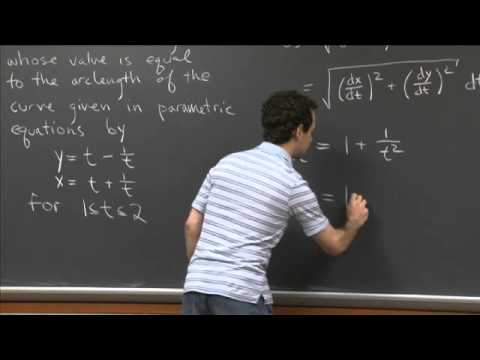#### Parametric Arclength | MIT 18.01SC Single Variable Calculus, Fall 2010

Topic: Calculus
Teacher: Joel Lewis
Concepts In This Lecture: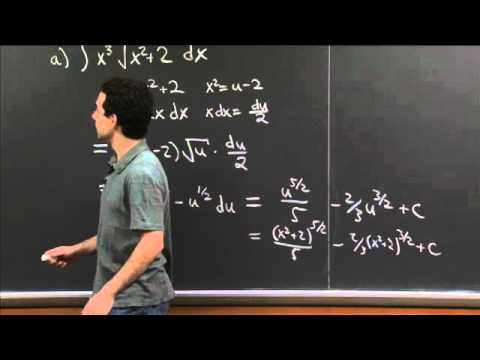#### Integration Practice III | MIT 18.01SC Single Variable Calculus, Fall 2010

Topic: Calculus
Teacher: Joel Lewis
Concepts In This Lecture: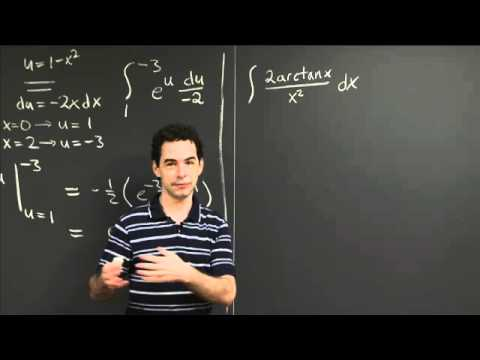#### Integration Practice IV | MIT 18.01SC Single Variable Calculus, Fall 2010

Topic: Calculus
Teacher: Joel Lewis
Concepts In This Lecture: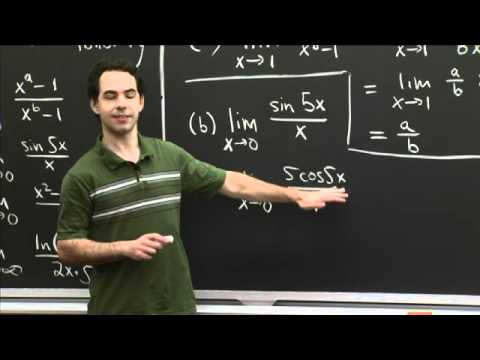#### l'Hopital Practice | MIT 18.01SC Single Variable Calculus, Fall 2010

Topic: Calculus
Teacher: Joel Lewis
Concepts In This Lecture: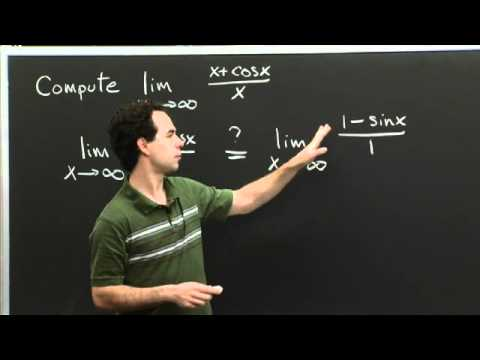#### Failure of L'Hospital's Rule | MIT 18.01SC Single Variable Calculus, Fall 2010

Topic: Calculus
Teacher: Joel Lewis
Concepts In This Lecture: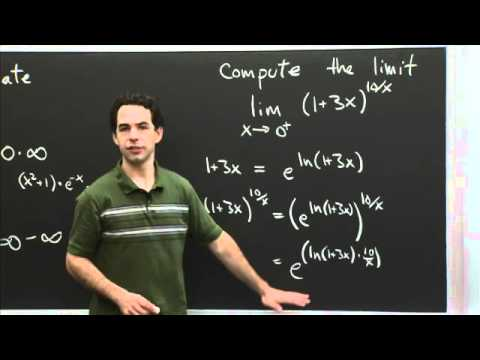#### Indeterminate forms | MIT 18.01SC Single Variable Calculus, Fall 2010

Topic: Calculus
Teacher: Joel Lewis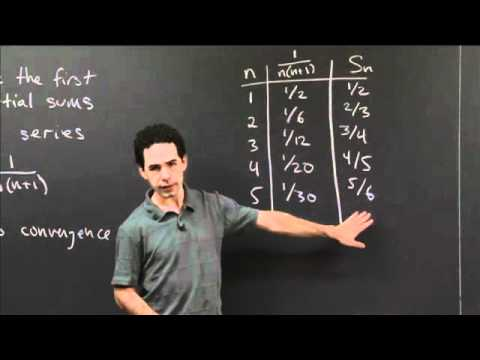#### Limit of a Series | MIT 18.01SC Single Variable Calculus, Fall 2010

Topic: Calculus
Teacher: Joel Lewis
Concepts In This Lecture: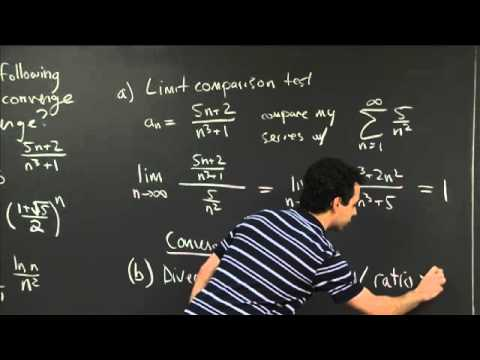#### Comparison Tests | MIT 18.01SC Single Variable Calculus, Fall 2010

Topic: Calculus
Teacher: Joel Lewis
Concepts In This Lecture: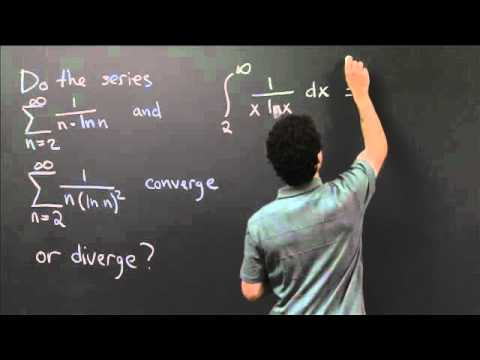#### Integral Test | MIT 18.01SC Single Variable Calculus, Fall 2010

Topic: Calculus
Teacher: Joel Lewis
Concepts In This Lecture: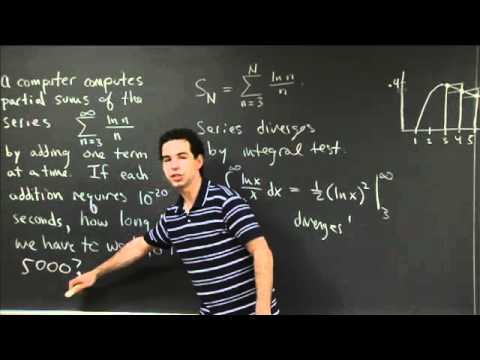#### Integral Test as Estimation | MIT 18.01SC Single Variable Calculus, Fall 2010

Topic: Calculus
Teacher: Joel Lewis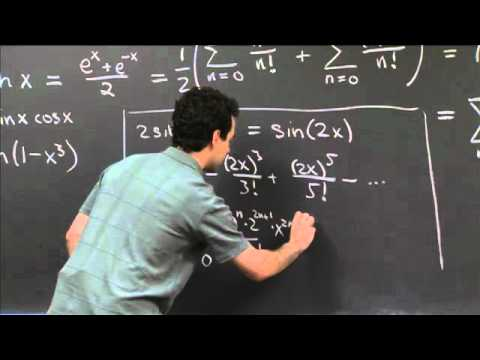#### Finding Taylor's Series | MIT 18.01SC Single Variable Calculus, Fall 2010

Topic: Calculus
Teacher: Joel Lewis

#### Our Philosophy

TeachingTree is an open platform that lets anybody organize educational content. Our goal is for students to quickly access the exact clips they need in order to learn individual concepts. Everyone is encouraged to help by adding videos or tagging concepts. Read our about page for more info.

#### Talk To Us

If you have any suggestions for how to make the TeachingTree better, questions about how to use the site, or just want to chat, email whatsup@teachingtree.co and we'll get back to you. We're always happy to talk.